M403K Final Exam Solutions
May 10, 2002

Problem 1. Graphing

Consider the function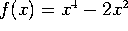.

a) Find the partition points of f and make a sign chart for f.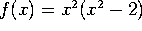, so the partition points are 0,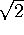and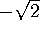. The function is positive for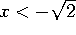and for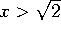. It is negative for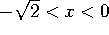and for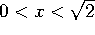.

b) Find the critical points of f and make a sign chart for f'.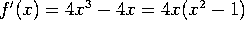, so the critical points are -1, 0 and 1. f' is negative for x<-1, positive for -1 < x < 0, negative for 0<x<1 and positive for x>1.

c) Find the inflection points of f and make a sign chart for f''.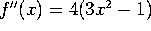, so the inflection points are at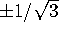. f'' is positive for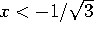and for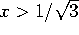, and negative for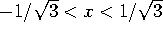.

d) On the back of this page, sketch the curve y=f(x). Mark all important points CLEARLY.

I can't draw this on screen, but the graph looks like a ``Mexican hat''. There are local minima at (-1,-1) and (1,-1), and a local maximum at (0,0). The graph is positive, decreasing and curving up for, then negative, decreasing and curving up for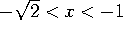, hitting a local minimum at (-1, -1). It is then negative, increasing, and curving up for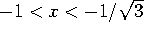, and is negative, increasing, and curving down for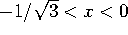, hitting a local maximum at (0,0). The remainder of the graph is a mirror image of the first half, since f(-x)=f(x).

Problem 2. Max-min

Consider the function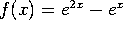.

a) Find all critical points of this function. For each one, say whether it is a local maximum, a local minimum, or neither.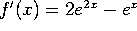. Setting this equal to zero we have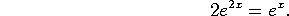But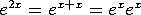, so dividing our equation by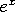gives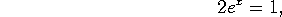so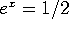, so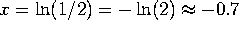. This is the ONLY critical point. Now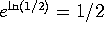, and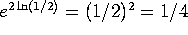, so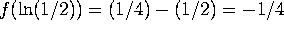. Now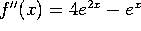, so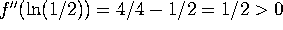, so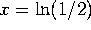is a local minimum.

b) Find the global maximum and minimum of f(x) in the interval [-5,5].

The candidates are the critical points and the endpoints.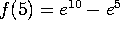is a huge positive number, and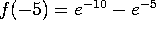is a tiny negative number, so the maximum is at x=5 and the minimum is at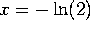.

Problem 3. Marginal analysis

The demand x for widgets is related to the price p by the demand equation x = 3000 - 100 p. The cost function is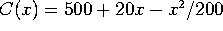.

a) Find the marginal cost, the marginal revenue, and the marginal profit at a production level of x=1200.

Solving for price in terms of demand gives p=30 - x/100, so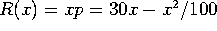. Since, we have a profit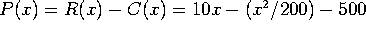. Taking derivatives we get our marginal quantities:

R'(x) = 30 - x/50, C'(x) = 2-=x/100, P'(x) = 10-x/100.

Finally, plugging in x=1200 gives R'(1200)=6, C'(1200)=8 and P'(1200)=-2. In other words, each additional widget costs us \$ 8, and only brings us \$ 6 in additional revenue, and so decreases our profit by \$ 2.

b) What is the production level that maximizes revenue? What production level maximizes profit?

To maximize revenue, set R'=0. This gives x=1500.

To maximize profit, set P'=0. This gives x=1000.

Problem 4. Exponential growth

An investor invests \$1000 at 7% interest, compounded continuously.

a) How much money will he have in 20 years? Express your answer as an exact expression (e.g. something like \$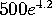- no, that's not the right answer), and then approximate it numerically (e.g., \$16,000).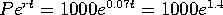. Now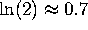(the Law of 70, remember?), so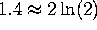and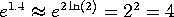. Thus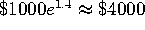.

b) When will there be \$10,000 in his account? Express your answer as an exact expression. You do NOT need to approximate it numerically.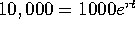, so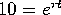, so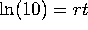, so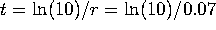. (This is a little under 33 years).

Problem 5. Rates of change

A quantity y is changing at a rate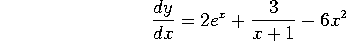When x=0, y=5. What does y equal when x=2?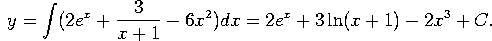Plugging in y(0)=5 we have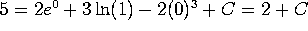, so C=3. Thus: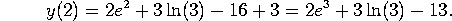Problem 6. Volume

A silo-shaped region is obtained by taking the region between the curve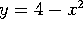and the x-axis and rotating it about the y-axis. [See figure]. We compute the volume of this region by slicing it into a stack of disks.

Note: This is a straightforward application of the ``slice and dice principle'', but doesn't directly correspond to any formulas in the book. I'm quite disappointed that only 4 or 5 people in the entire class attempted this problem.

a) Find the (approximate) radius and (approximate) volume of a disk at height y and thickness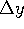.

The radius of the disk is just the value of x on the curve. Since, we have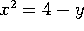, so our radius is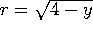. The area is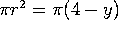, and the volume is (area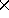thickness) =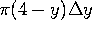.

b) By summing the answer to (a) and taking a limit, express the volume of the silo as a definite integral.

The sum of volumes is of the form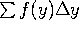, where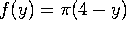, and y ranges from 0 to 4. Taking the limit as the number of slices goes to infinity gives the definite integral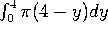.

c) Evaluate this integral to get the total volume.

The integral evaluates to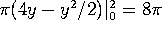.

Part II:

Evaluate the following. The limits and integrals should be simplified as much as possible, but you don't have to simplify the derivatives:

a)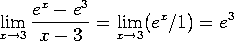b)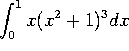. Set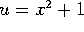.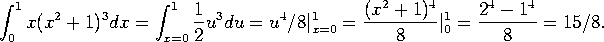c)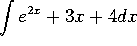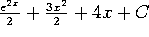.

d)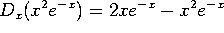.

e) f'(x), where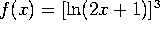. Apply the chain rule twice to get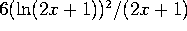f)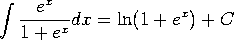. This comes from the substitution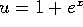.

g) dy/dx, where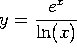is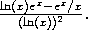h)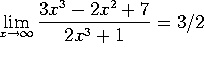. (Either apply L'Hopital 3 times, or divide top and bottom by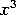and take a limit).

i)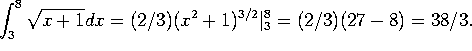Note that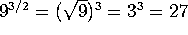and that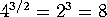.

j)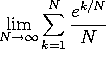. This sum is of the form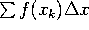, where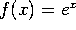and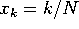. Taking the limit gives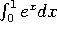, which works out to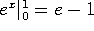.